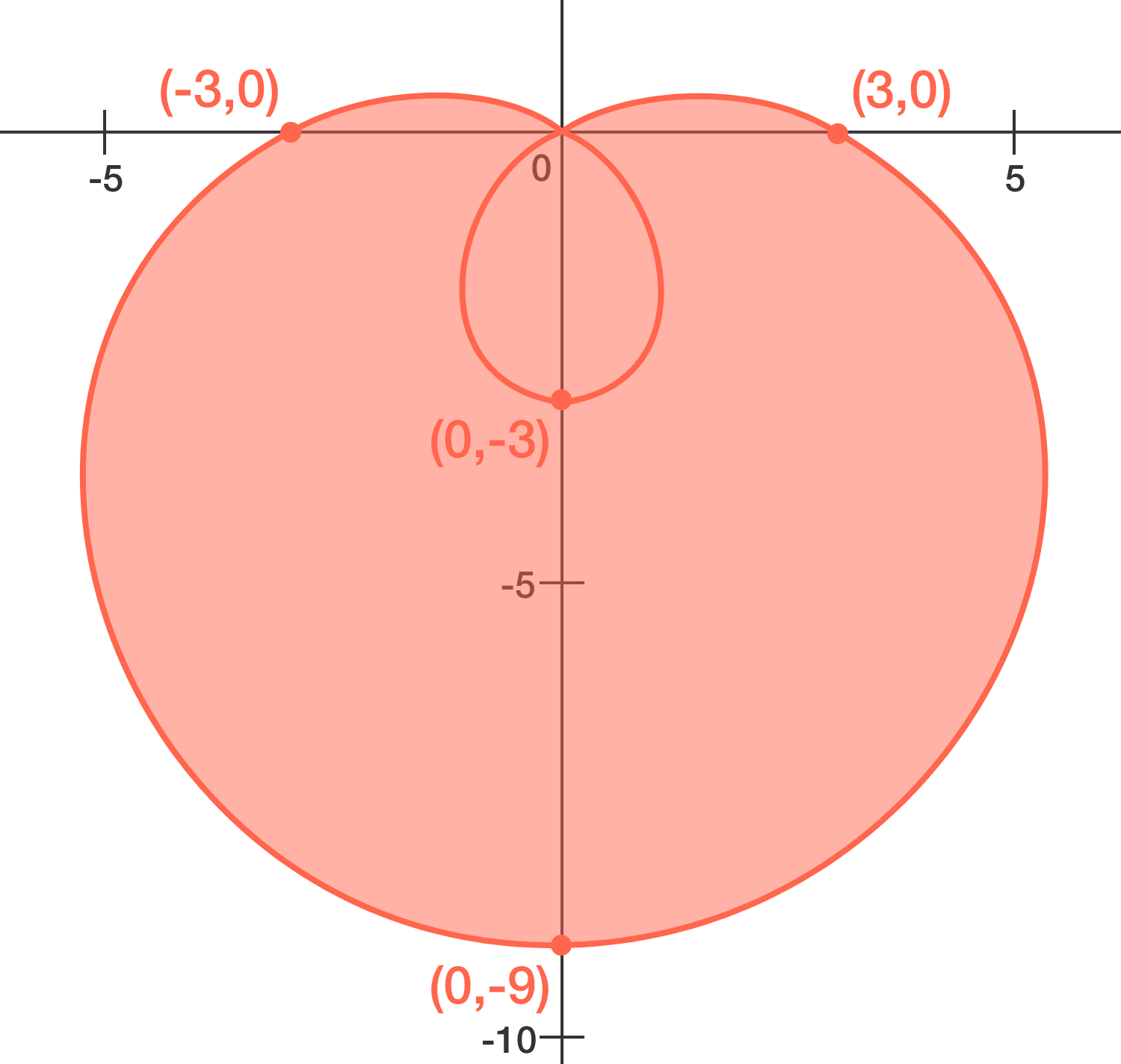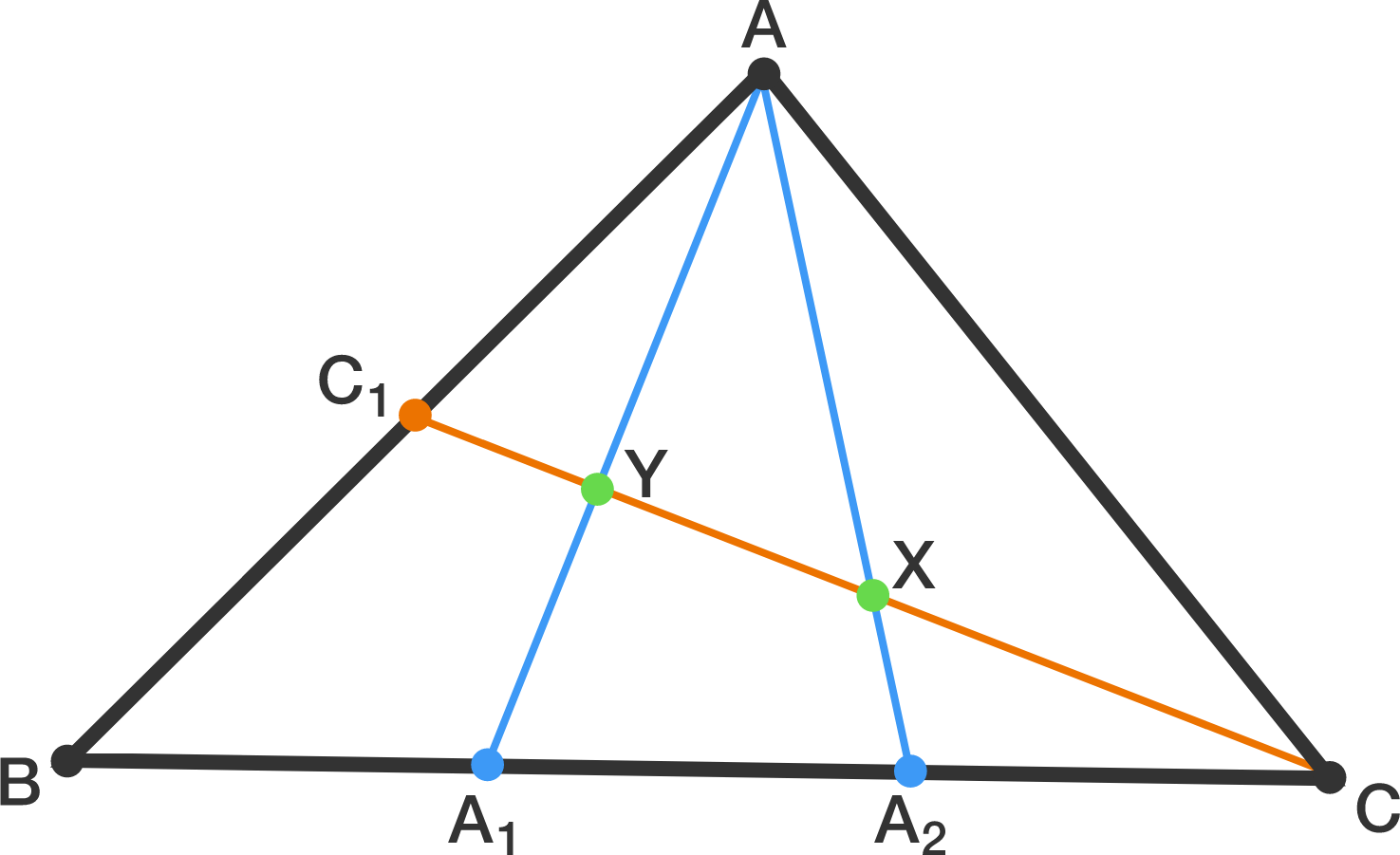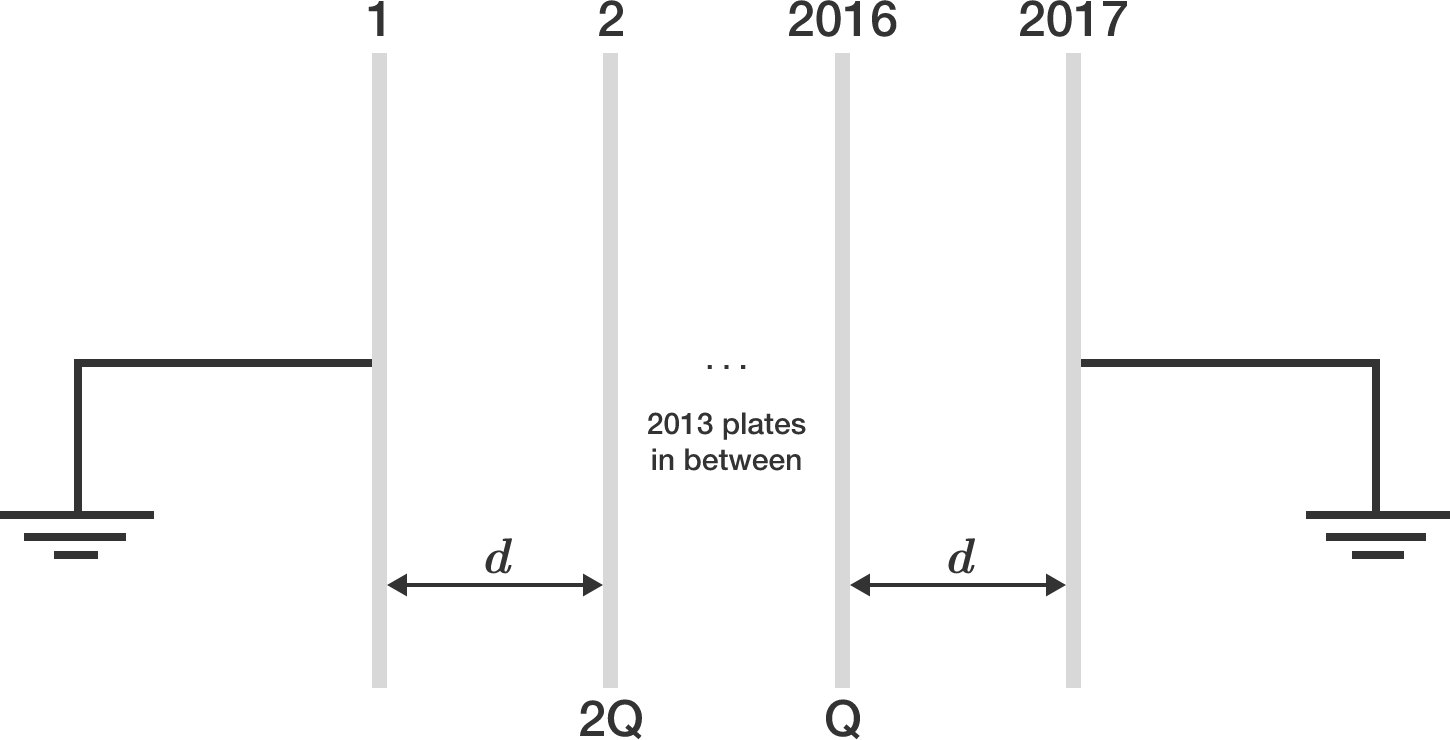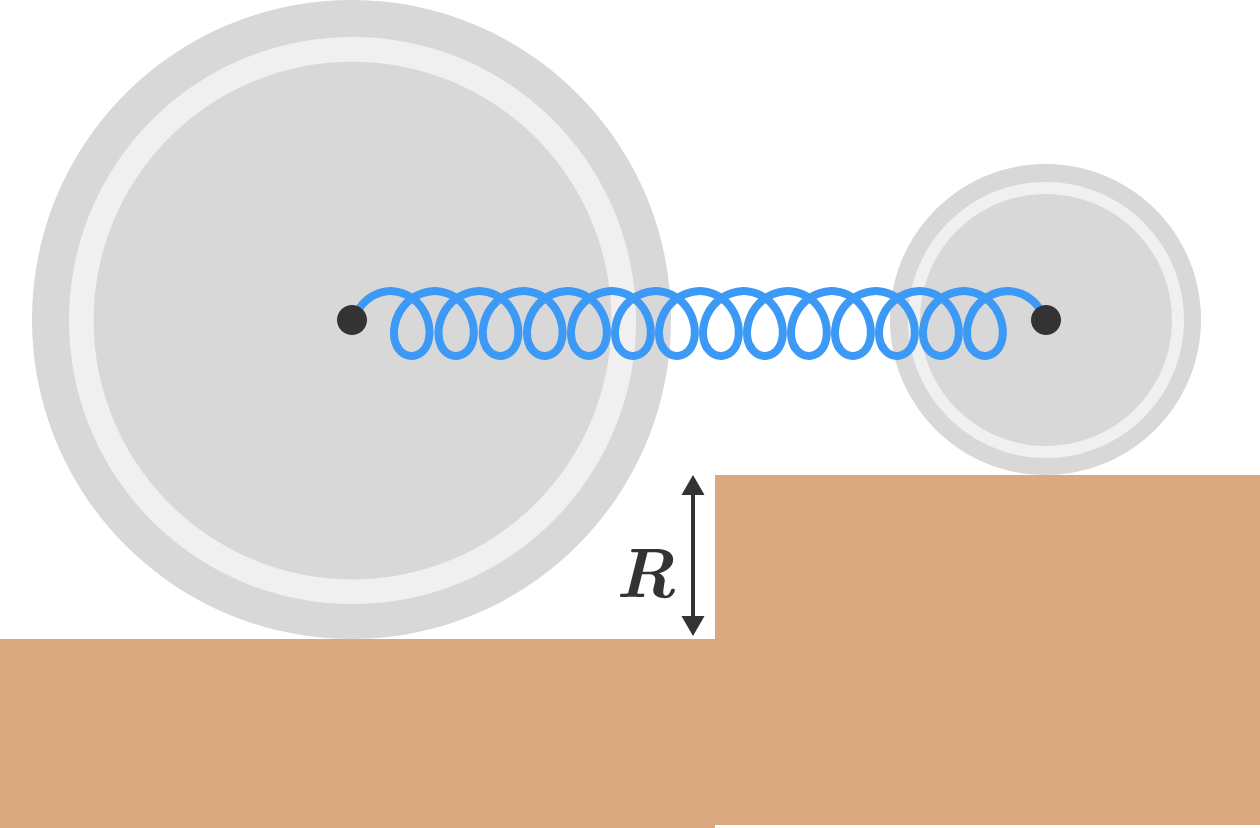# Problems of the Week

Contribute a problem

# 2017-05-22 AdvancedFor Mother's Day, Little Albert wants to show just how much he loves his mom by making a card for her with a big, colorful heart on the front. He gets out his paper, uncaps a marker, and gets to work.

The curve that Little Albert drew is called a limaçon. (The extra loop inside the heart will be invisible to his mom after coloring.) The polar equation for the particular limaçon that he drew is $r = 3 - 6 \sin \theta.$ The area of the region that Little Albert colored in is equal to $a \pi + \frac{b \sqrt{3}}{c},$ where $a,$ $b,$ and $c$ are positive integers, and $b$ and $c$ are coprime.

Find $a + b + c.$In the diagram above, suppose that $BA_{1} = A_1A_2 = A_2C$ and $AC_1 = C_1B$. If $C_1Y = 2017$, then $XY = \frac{p}{q}$, where $p$ and $q$ are coprime positive integers. Find $p + q$.2017 identical metallic plates (initially uncharged), each having area of cross section $A$, are each separated by a distance of $d,$ as shown in the figure above.

Plates 2 and 2016 are given charges $2\mathbf Q$ and $\mathbf Q,$ respectively. Plates 1 and 2017 are both earthed via a very thin conducting wire.

If the potential difference between plates 1728 and 1729 is of the form $\dfrac{a Qd}{bA\epsilon_0}$, where $a$ and $b$ are coprime positive integers, then submit your answer as $a+b$.


Details and Assumptions:

• $\epsilon_0$ denotes the permittivity of free space.
• The plates are very large, and the phenomena of fringing of electric field lines are neglected.
• Because of the large size, the electric field due to any particular plate is considered as that of an infinitely large, thin conducting plate.

Let $f(x) = x + e^x - 1$, and let $f^{-1} (x)$ denote the inverse function of $f(x)$.

Then the integral $\displaystyle \int_{e}^{1 + e^2} f^{-1} (x) \, dx$ evaluates to $k_0 + k_1 e^1 + k_2 e^2 + \cdots + k_n e^n ,$ where $k_0, k_1, \ldots, k_n$ are rational numbers and $e\approx 2.718$ is Euler's number.

If the sum $k_0 + k_1 + k_2 + \cdots + k_n$ can be expressed as $\frac{A}{B},$ where $A$ and $B$ are coprime positive integers, what is $A+B+n?$Two uniform circular disks of masses $M$ and $4M$ and radii $R$ and $2R,$ respectively, are connected by a massless spring of force constant $k$ and placed at different heights so that the spring is parallel to the horizontal, as shown above.

Initially, the spring is compressed, and then the system is released from rest. Assuming friction is large enough to prevent slipping, find the angular frequency of oscillation of the system.

If you answer comes in the form of $\sqrt{ \dfrac{ak}{bM}},$ where $a$ and $b$ are coprime positive integers, then enter $a+b$ as the final answer.

×

Problem Loading...

Note Loading...

Set Loading...